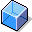Formula ParameterA formula is identified by its formula key. It is composed of formula parameters that are identified by parameter IDs.

You create formula parameters and formulas in Customizing for work centers:

• Formula parameter in Define formula parameters for work centers
• Formulas in Define formulas for work centers

You assign each formula parameter to a data field in the work center or routing. When the formula is evaluated, each formula parameter has the value that you have entered in the corresponding data field.

The Origin of Formula Parameter Values

You must enter the origin of every formula parameter in Customizing. The origin controls the assignment of formula parameters to data fields. According to its origin a formula parameter is either automatically assigned to a data field or you have to enter a field name as well as the origin.

You can refer to formula parameters in work centers or routings either by the parameter ID or by the key word, which you have to assign to every formula parameter in Customizing. This key word appears with the assigned field in the work center or routings screen.

Formula parameter values can have the following origins:

• Standard value

With the help of the standard value key you can assign up to six formula parameters for standard values to a work center and consequently to an operation in a routing.

• Formula constants in the work center

You can assign a constant value and a unit to up to six work center constants.

• General formula constants

You assign general formula constants a fixed value and an unit in Customizing for Formula parameters

• General operation value

The formula parameter is linked to a data field in the general operation data. Typical fields are:

• Lot size
• Base quantity
• Number of splits (partial lots)
• Number of employees

If formula parameters have this origin, you must enter the corresponding field names in Customizing.

• User-defined field in the operation

The value of a user-defined field in the operation is assigned to the formula parameter.

In this case the user-field key also controls the assignment of the formula parameter to the data field by making two data fields available for formula parameters. You define user-field keys in Customizing Routings under Create user-defined fields The key words, with which the data fields are labeled in the operation, are not defined in the formula parameter directly, but in the user-field key.

• Field in production resource/tool (PRT) assignment

You assign a data field in the production resource/tool assignment to the formula parameter, for example:

• the quantity of the PRT
• the usage value of the PRT
• Production resource/tool constants

You assign constant values to the formula parameters in the PRT master record.

These formula parameters can only be used in formulas for PRT management.